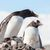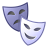## Dual OV5640 cameras on iMX8M Plus

cancel
Showing results for
Did you mean:

## Dual OV5640 cameras on iMX8M Plus

382 ViewsSenior Contributor I

Dear iMX experts,

I'm trying to enable the second MPI-CSI interface of iMX8MP to use a pair of OV5640 cameras (in RAW10 mode). Hereafter my .dts :

``````+++ b/arch/arm64/boot/dts/freescale/imx8mp-evk-ov5640-raw.dts
@@ -0,0 +1,158 @@
+
+#include "imx8mp-evk.dts"
+
+
+&iomuxc {
+	pinctrl_csi1_pwn: csi1_pwn_grp {
+		fsl,pins = <
+			MX8MP_IOMUXC_SAI1_RXC__GPIO4_IO01       0x19
+		>;
+	};
+
+	pinctrl_csi1_rst: csi1_rst_grp {
+		fsl,pins = <
+			MX8MP_IOMUXC_SAI1_RXFS__GPIO4_IO00      0x19
+		>;
+	};
+};
+
+&i2c2 {
+	/delete-node/ov5640_mipi@3c;
+
+	ov5640_raw_0: ov5640_raw_mipi@3c {
+		compatible = "ovti,ov5640_raw";
+		reg = <0x3c>;
+		pinctrl-names = "default";
+		pinctrl-0 = <&pinctrl_csi0_pwn>, <&pinctrl_csi0_rst>, <&pinctrl_csi_mclk>;
+		clocks = <&clk IMX8MP_CLK_IPP_DO_CLKO2>;
+		clock-names = "xclk";
+		assigned-clocks = <&clk IMX8MP_CLK_IPP_DO_CLKO2>;
+		assigned-clock-parents = <&clk IMX8MP_CLK_24M>;
+		assigned-clock-rates = <24000000>;
+		csi_id = <0>;
+		powerdown-gpios = <&gpio2 11 GPIO_ACTIVE_HIGH>;
+		reset-gpios = <&gpio1 6 GPIO_ACTIVE_LOW>;
+		mclk = <24000000>;
+		mclk_source = <0>;
+		mipi_csi;
+		status = "okay";
+
+		port {
+			ov5640_raw_mipi_0_ep: endpoint {
+				remote-endpoint = <&mipi_csi0_ep>;
+				data-lanes = <1 2>;
+				clock-lanes = <0>;
+			};
+		};
+	};
+};
+
+&i2c3 {
+	clock-frequency = <100000>;
+	pinctrl-names = "default";
+	pinctrl-0 = <&pinctrl_i2c3>;
+	status = "okay";
+
+	/delete-node/ov5640_mipi@3c;
+
+	ov5640_raw_1: ov5640_raw_mipi@3c {
+		compatible = "ovti,ov5640_raw";
+		reg = <0x3c>;
+		pinctrl-names = "default";
+		pinctrl-0 = <&pinctrl_csi1_pwn>, <&pinctrl_csi1_rst>/*, <&pinctrl_csi_mclk>*/; Commented out &pinctrl_csi_mclk
+		clocks = <&clk IMX8MP_CLK_IPP_DO_CLKO2>;
+		clock-names = "xclk";
+		assigned-clocks = <&clk IMX8MP_CLK_IPP_DO_CLKO2>;
+		assigned-clock-parents = <&clk IMX8MP_CLK_24M>;
+		assigned-clock-rates = <24000000>;
+		csi_id = <1>;
+		powerdown-gpios = <&gpio4 1 GPIO_ACTIVE_HIGH>;
+		reset-gpios = <&gpio4 0 GPIO_ACTIVE_LOW>;
+		mclk = <24000000>;
+		mclk_source = <0>;
+		mipi_csi;
+		status = "okay";
+
+		port {
+			ov5640_raw_mipi_1_ep: endpoint {
+				remote-endpoint = <&mipi_csi1_ep>;
+				data-lanes = <1 2>;
+				clock-lanes = <0>;
+			};
+		};
+	};
+};
+
+
+&mipi_csi_0 {
+	status = "okay";
+
+	port@0 {
+		reg = <0>;
+		mipi_csi0_ep: endpoint {
+			remote-endpoint = <&ov5640_raw_mipi_0_ep>;
+			data-lanes = <2>;
+			csis-hs-settle = <13>;
+			csis-clk-settle = <2>;
+			csis-wclk;
+		};
+	};
+};
+
+&mipi_csi_1 {
+	status = "okay";
+
+	port@1 {
+		reg = <1>;
+		mipi_csi1_ep: endpoint {
+			remote-endpoint = <&ov5640_raw_mipi_1_ep>;
+			data-lanes = <2>;
+			csis-hs-settle = <13>;
+			csis-clk-settle = <2>;
+			csis-wclk;
+		};
+	};
+};
+
+	status = "okay";
+};
+
+&isi_0 {
+	status = "okay";
+
+	cap_device {
+		status = "okay";
+	};
+
+	m2m_device {
+		status = "disabled";
+	};
+};
+
+&isi_1 {
+	status = "okay";
+
+	cap_device {
+		status = "okay";
+	};
+};
+
+&dewarp {
+	status = "okay";
+};``````

From the EVK schematic, I observed that both MIPI-CSI camera interfaces share the the same CSI_nRST and CSI_MCLK signal, and if I don't comment out <&pinctrl_csi_mclk> in the following line of 2nd node of ov5640 :

``+		pinctrl-0 = <&pinctrl_csi1_pwn>, <&pinctrl_csi1_rst>, <&pinctrl_csi_mclk>;``

I will get following error :

``````[ 2.745247] imx8mp-pinctrl 30330000.pinctrl: pin MX8MP_IOMUXC_GPIO1_IO15 already requested by 1-003c; cannot claim for 2-003c
[ 2.756578] imx8mp-pinctrl 30330000.pinctrl: pin-20 (2-003c) status -22
[ 2.763205] imx8mp-pinctrl 30330000.pinctrl: could not request pin 20 (MX8MP_IOMUXC_GPIO1_IO15) from group csi_mclk_grp on device 30330000.pinctrl
[ 2.776430] ov5640_raw 2-003c: Error applying setting, reverse things back
[ 2.783342] ov5640_raw: probe of 2-003c failed with error -22``````

However, if I comment out <&pinctrl_csi_mclk> :

``+		pinctrl-0 = <&pinctrl_csi1_pwn>, <&pinctrl_csi1_rst>/*, <&pinctrl_csi_mclk>*/; Commented out &pinctrl_csi_mclk``

Then it seems that there's issue reading the 2nd OV5640 sensor via I2C :

``````[    2.610614] ov5640_raw 1-003c: 1-003c supply DOVDD not found, using dummy regulator
[    2.618341] ov5640_raw 1-003c: 1-003c supply AVDD not found, using dummy regulator
[    2.625989] ov5640_raw 1-003c: 1-003c supply DVDD not found, using dummy regulator
...
[    2.698674] ov5640_raw 2-003c: 2-003c supply DOVDD not found, using dummy regulator
[    2.706389] ov5640_raw 2-003c: 2-003c supply AVDD not found, using dummy regulator
[    2.714001] ov5640_raw 2-003c: 2-003c supply DVDD not found, using dummy regulator
[    2.766549] ov5640_raw 2-003c: ov5640_read_reg: error: reg=300a
[    2.772489] ov5640_raw 2-003c: ov5640_check_chip_id: failed to read chip identifier``````

Note that ov5640_raw 1-003c and ov5640_raw 2-003c are 2 instances of the same driver : ov5640_raw. And the 1st one seems to be recognized and work :

``````# v4l2-ctl -d /dev/video0 --set-fmt-video=width=1280,height=720,pixelformat='BG10' --stream-mmap --stream-count=500
[   33.697582] bypass csc
[   33.699990] input fmt YUV4
[   33.702722] output fmt BG10
[   33.758159] can1-stby: disabling
[   33.761393] can2-stby: disabling
<<<<<<<<<<<<<<<<<<<<<<<<<< 24.28 fps
<<<<<<<<<<<<<<<<<<<<<<<< 24.28 fps
<<<<<<<<<<<<<<<<<<<<<<<< 24.28 fps
<<<<<<<<<<<<<<<<<<<<<<<<< 24.28 fps
<<<<<<<<<<<<<<<<<<<<<<<< 24.28 fps
<<<<<<<<<<<<<<<<<<<<<<<< 24.28 fps
<<<<<<<<<<<<<<<<<<<<<<<< 24.28 fps
<<<<<<<<<<<<<<<<<<<<<<<<< 24.28 fps
<<<<<<<<<<<<<<<<<<<<<<<< 24.28 fps
<<<<<<<<<<<<<<<<<<<<<<<< 24.28 fps
<<<<<<<<<<<<<<<<<<<<<<<<< 24.28 fps
<<<<<<<<<<<<<<<<<<<<<<<< 24.28 fps``````

I wonder if I would need to modify the EVK hardware to re-route the reset, powerdown GPIOs as well as the MCLK signals for the 2nd camera interface, please ?

Thanks in advance and best regards,
Khang

5 Replies
262 ViewsContributor II

Does it mean that we can't use both the camera interfaces? Is there a way around it? I am trying to implement picture in picture.

258 ViewsSenior Contributor I

Hi @avijitnsec ,

You could work-around on the hardware to separate the RST/PWDN pair which are mainly for the first ov5640 and re-wire some spare GPIOs as second RST/PWDN pair for second ov5640 and modify the dts according to that.

I switched to use customized cameras that do not need RST/PWDN signals.

Br, Khang

371 ViewsNXP TechSupport

Hi Khang

RST/PWN pins for 2 camera ports are being shared so the default BSP

cannot support 2 ov5640 cameras, sorry.

Best regards
igor

366 ViewsSenior Contributor I

Hi Igor,

Thanks for your prompt reply. I think that I will modify the EVK hardware to separate the RST/PWDN signals for each camera. But do you think that I need to separate also MCLK signal ?

Thanks,
K

355 ViewsNXP TechSupport

I believe there is no special need to separate also MCLK signal.

Best regards
igor